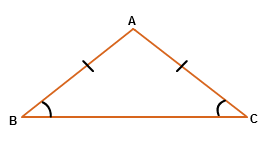# LIKTRI Editorial

Practice

Author: Mann Mehta

Tester: Jaymeet Mehta

Editorialist: Vatsal Parmar

Easy

# PREREQUISITES

Basic Math, Triangle Properties, Geometry

# PROBLEM

You are given a \$9-Queries\$ on an Isosceles Triangle, in which you get to know isosceles sides and any one angle of an Isosceles Triangle. Your task is to predict other two angles of Isosceles Triangle.

# EXPLANATION

Here there are total 3-ways such that we select 2 side as an isosceles side in a triangle. That's why total 9-queries are possible for each angel with all 3 ways.(3-Angles × 3-Ways &equals; 9-Queries)

What is Isosceles Triangle ?

In geometry, an isosceles triangle is a triangle that has at least two sides of equal length.ang(A)+ang(B)+ang(C)=180
If AB &equals; AC then angle(B) &equals; angle(C)

Now, we go through first three queries Where \$AB\$ and \$AC\$ are isosceles side therefore \$angle(B) &equals; angle(C)\$ .

1) Query-1

Let’s consider angle(A) which is given as A and suppose other two angles angle(B) and angle(C) as X.

Now applying the angle sum property.

angle(A)+angle(B)+angle(C)=180
A &plus; X &plus; X &equals; 180
2X &equals; 180 − A
X &equals; (180 − A) / 2

2) Query-2
Let's consider \$angle(B)\$ which is given as \$B\$ and suppose other two angles \$angle(A)\$ as \$X\$ and \$angle(C)\$ as \$Y\$.
Now applying the angle sum property.

angle(A)+angle(B)+angle(C)=180
X &plus; B &plus; Y &equals; 180
We know that angle(B) &equals; angle(C) therefore angle(C)=B
X &plus; B &plus; B &equals; 180
X &plus; 2B &equals; 180
X &equals; 180 − 2B

Now in this query angle(B) and angle© is an isosceles angle’s therefore to make a valid triangle it’s value must be in range :- 0 < angle(B),angle© < 90

3) Query-3

Let’s consider angle(C) which is given as C and suppose other two angles angle(A) as X and angle(B) as Y.

Now applying the angle sum property.

angle(A)+angle(B)+angle(C)=180
X &plus; Y &plus; C &plus; &equals; 180
We know that angle(B) &equals; angle(C) therefore angle(B)=C
X &plus; C &plus; C &plus; &equals; 180
X &plus; 2C &equals; 180
X &equals; 180 − 2C

Now in this query angle(B) and angle© is an isosceles angle’s therefore to make a valid triangle it’s value must be in range :- 0 < angle(B),angle© < 90

Now similarly go for other queries also.

#TIME COMPLEXITY
Time Complexity is O(1) per test case.

#SOLUTIONS